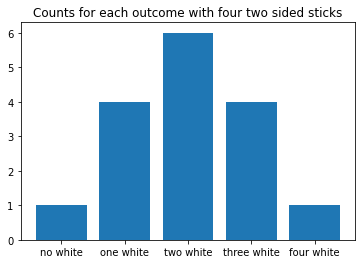### Plotting Distributions¶

The fourth row of our sennet table contained the values:

$$1 ~ 4 ~ 6 ~ 4 ~ 1$$

We can draw a plot of this using bars to represent the counts of each outcome.

In [ ]:
bars = ['no white', 'one white', 'two white', 'three white', 'four white']
counts = [1, 4, 6, 4, 1]

In [ ]:
plt.bar(bars, counts)
plt.title('Counts for each outcome with four two sided sticks');Using this plot, we come back to the problem of determining the probability of a given outcome or outcomes. Here, we can interpret this probability as the relative area of a given bar to the overall count. For example, we consider each bar having width of one unit, and height of the count. Thus, we have a total area of:

$$\textbf{TOTAL AREA} = 1 + 4 + 6 + 4 + 1 = 16$$

This is the total number of possible outcomes. Thus, determining the probability of a specific outcome is as simple as dividing the total area of our bars by the area under the event of interest.

$$P(\text{two white}) = \frac{\text{area of bar for two white}}{\text{total area}} = \frac{6}{16}$$

### Problems¶

1. Use the plot above to determine the probability of zero white sticks.
2. Use the plot above to determine the probability of one white stick?
3. What is the probability of one, two, or three white sticks and how do we use the graph to determine this.